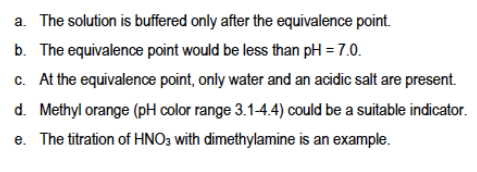# Problem: Consider the titration of a strong acid with a weak base. Which of the following statements is incorrect?

###### FREE Expert Solution
90% (439 ratings)
###### Problem Details

Consider the titration of a strong acid with a weak base. Which of the following statements is incorrect?Frequently Asked Questions

What scientific concept do you need to know in order to solve this problem?

Our tutors have indicated that to solve this problem you will need to apply the Equivalence Point concept. If you need more Equivalence Point practice, you can also practice Equivalence Point practice problems.

What is the difficulty of this problem?

Our tutors rated the difficulty ofConsider the titration of a strong acid with a weak base. Wh...as medium difficulty.

How long does this problem take to solve?

Our expert Chemistry tutor, Jules took 3 minutes and 10 seconds to solve this problem. You can follow their steps in the video explanation above.

What professor is this problem relevant for?

Based on our data, we think this problem is relevant for Professor Freeman's class at SCAR.